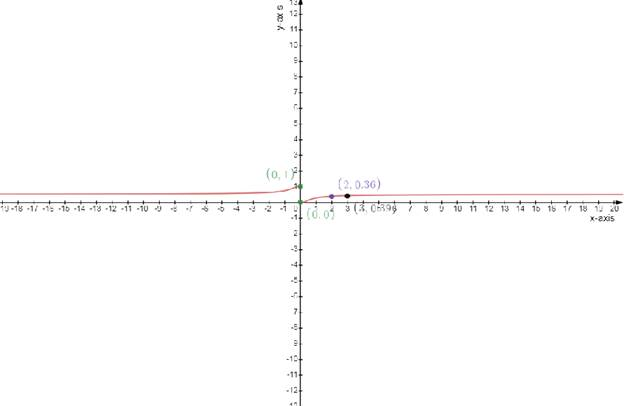# To graph: the given function f to estimate the maximum and minimum values.### Single Variable Calculus: Concepts...

4th Edition
James Stewart
Publisher: Cengage Learning
ISBN: 9781337687805### Single Variable Calculus: Concepts...

4th Edition
James Stewart
Publisher: Cengage Learning
ISBN: 9781337687805

#### Solutions

Chapter 4, Problem 20RE

(a)

To determine

## To graph:the given function f to estimate the maximum and minimum values.

Expert Solution

### Explanation of Solution

Given:

The function is

f(x)=11+e1x

Concept used:

The slope of the tangent to a curve f(x) at any point a is given by the value of the first derivative of the slope at the point x=a .

The tangent to be horizontal so the slope should be equal to 0

That is dydx=0 .

Calculation:

The function is

f(x)=11+e1x................................(1)

Draw the table

f(x)=11+e1x

Test one point in each of the region formed by the graph

If the point satisfies the function then shade the entire region to denote that every point in the region satisfies the function

 x−axis 0 0 2 3 y−axis 0 1 0.36 0.39

Draw the graph

f(x)=11+e1x(b)

To determine

Expert Solution

f'(0)=

### Explanation of Solution

Given:

The function f(x)=11+e1x

Concept used:

The definition of a vertical cusp is that the one sided limits of the derivative approach opposite ±

: positive infinity on one side and negative infinity on the other side . A vertical tangent has the one sided limits of the derivative equal to the same sign of infinity

The derivative at the relevant point is undefined in both the cusp and the vertical tangent

Calculation:

The function is f(x)=11+e1x.........(1)

Differentiating equation (1) with respect to x

f(x)=11+e1xf(x)=e1xx2(1+e1x)2

f(x) is the maximum value

(c)

To determine

Expert Solution

f'(0)=

### Explanation of Solution

Given:

The function f(x)=11+e1x

Concept used:

The definition of a vertical cusp is that the one sided limits of the derivative approach opposite ±

: positive infinity on one side and negative infinity on the other side . A vertical tangent has the one sided limits of the derivative equal to the same sign of infinity

The derivative at the relevant point is undefined in both the cusp and the vertical tangent

Calculation:

The function is f(x)=11+e1x.........(1)

Differentiating equation (1) with respect to x

f(x)=11+e1xf(x)=e1xx2(1+e1x)2

f(x) is the maximum value

f'(0)=

The vertical tangent means that the derivative at that point approaches infinity

Since the slope is infinitely large

(d)

To determine

Expert Solution

f'(0)=

### Explanation of Solution

Given:

The function f(x)=11+e1x

Concept used:

The definition of a vertical cusp is that the one sided limits of the derivative approach opposite ±

: positive infinity on one side and negative infinity on the other side . A vertical tangent has the one sided limits of the derivative equal to the same sign of infinity

The derivative at the relevant point is undefined in both the cusp and the vertical tangent

Calculation:

The function is f(x)=11+e1x.........(1)

Differentiating equation (1) with respect to x

f(x)=11+e1xf(x)=e1xx2(1+e1x)2................................(2)

f(x) is the maximum value Again differentiating equation (2) with respect to x

f=e1xx2(1+e1x)2f=(e1x)2x4(1+e1x)3

f(x) is the maximum value

f(0)=

The vertical tangent means that the derivative at that point approaches infinity

Since the slope is infinitely large

(e)

To determine

Expert Solution

f(0)=

### Explanation of Solution

Given:

The function f(x)=11+e1x

Concept used:

The definition of a vertical cusp is that the one sided limits of the derivative approach opposite ±

: positive infinity on one side and negative infinity on the other side . A vertical tangent has the one sided limits of the derivative equal to the same sign of infinity

The derivative at the relevant point is undefined in both the cusp and the vertical tangent

Calculation:

The function is f(x)=11+e1x.........(1)

Differentiating equation (1) with respect to x

f(x)=11+e1xf=(e1x)2x4(1+e1x)30=(e1x)2x4(1+e1x)3(e1x)2=0.............................(2)

f(0)=

The vertical tangent means that the derivative at that point approaches infinity

Since the slope is infinitely large

### Have a homework question?

Subscribe to bartleby learn! Ask subject matter experts 30 homework questions each month. Plus, you’ll have access to millions of step-by-step textbook answers!